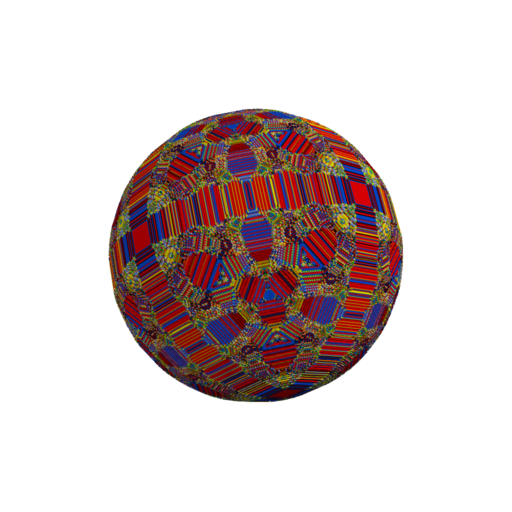This document gives an introduction to computing and visualizing various Abelian networks in Julia.

For a tutorial on installing Julia and setting up Jupyter notebooks see here. For a general introduction to Julia from the viewpoint of Linear Algebra, check out this page.

In :
using Plots # for visualization to install type in ] add Plots


# Abelian Sandpile¶

In :
#######################
## Description
##		computes the stabilization of the 2-dimensional
##		sandpile on a square
## Inputs
## 		S: an array of integers specifying the initial condition
## Output
##      returns odometer, T, sandpile S is stabilized in place

function stabilize!(S)
#initialize the all 0 odometer
T = fill(0, size(S))

#iterate over all sites and try to topple
#until it is not possible anymore
isTopple = true;
while(isTopple)
isTopple = false
for y in 1:size(S,2)
for x in 1:size(S,1)
isTopple = isTopple | topple!(S,T,x,y)
end
end
end

return T;
end

## fire a single site in S once
## i.e. increment odometer
## remove chips from S[x,y]
## and give chips to neighbors
## (x+1,y), (x-1,y), (x,y+1), (x,y-1)
function topple!(S,T,x,y)
#number of times fire site (x,y)
#we take max in case there is a hole at (x,y)
z = max(floor(S[x,y]/4), 0)
#increment the odometer
T[x,y]+=z
S[x,y]-=4*z;

#give sand to each neighbor
if(x > 1)
S[x-1,y]+=z
end
if(x < size(S,1))
S[x+1,y]+=z
end
if(y > 1)
S[x,y-1]+=z
end
if(y < size(S,2))
S[x,y+1]+=z
end

#if we have toppled return true
#else return false
if(z > 0)
return true;
else
return false
end
end

Out:
topple! (generic function with 2 methods)
##### Abelian sandpile examples¶

We compute several examples of Abelian sandpiles using the above function.

In :
N = 50
S = fill(4,N,N)
T = stabilize!(S)
heatmap(S)

Out:
In :
N = 200
S = fill(1,N,N)
S[div(N,2),div(N,2)]+=N^2
T = stabilize!(S); #will take a couple of seconds
S[T.==0].=-1;
heatmap(S)

Out:
In :
N = 200
S = rand(0:1,N,N)
init_condition = copy(S)
S[div(N,2),div(N,2)]+=N^2
T = stabilize!(S) #will take a couple of seconds
S = S .- init_condition
S[T.==0].=-1;
heatmap(S)

Out:
##### Efficient computation of symmetric Abelian sandpiles in arbitrary dimensions¶

If you have access to a GPU and want to compute large symmetric (e.g. single-source, constant, ...) sandpiles in $\mathbf{Z}^d$ see code here. This code uses symmetry of the initial condition to reduce to computing the sandpile on the simplex, $\mathcal{S}_M = \{ (x_1, \ldots, x_d) \in \mathbf{Z}^d | M \leq x_1 \leq \cdots \leq x_d \leq 1 \}$. In high dimensions, computing sandpiles on the simplex improves space complexity by a factor of $d^d$. This reduction in size also leads to a faster algorithm when using parallelization.

For example, an NVDIA RTX 2080 TI can compute the sandpile on $\mathbf{Z}^4$ started with $2^{30}$ chips at the origin in about a day:## Exploding sandpiles¶

We visualize exploding sandpiles via the parallel toppling procedure. First we modify the above code to perform parallel updates, then look at two examples of parallel toppling sandpiles.

In :
#######################
## Description
##		computes num_iter parallel topple updates of a sandpile on a square
## Inputs
## 		S: an array of integers specifying the sandpile
## 		T: an array of integers specifying current odometer
## 		num_iters: an integer specifying how many parallel update steps to take
## Output
##      sandpile S is updated in place

function parallel_topple!(S, T, num_iters)

for i in 1:num_iters
S_prev = copy(S)
for y in 1:size(S,2)
for x in 1:size(S,1)
one_topple!(S,S_prev,T,x,y)
end
end
end
end

## fire a single site in S once
## via the parallel update procedure
function one_topple!(S,S_prev, T,x,y)
## now topple according to how many
## grains it had at the PREVIOUS
## time step
z = max(floor(S_prev[x,y]/4), 0)

#number of times fire site (x,y)
#we take max in case there is a hole at (x,y)
#increment the odometer
T[x,y]+=z
S[x,y]-=4*z;

#give sand to each neighbor
if(x > 1)
S[x-1,y]+=z
end
if(x < size(S,1))
S[x+1,y]+=z
end
if(y > 1)
S[x,y-1]+=z
end
if(y < size(S,2))
S[x,y+1]+=z
end
end

Out:
one_topple! (generic function with 1 method)
In :
## start with all 4s everywhere in a square of side length 200
## and parallel topple for (25)^2 time steps
N = 100
S = fill(4,N,N)
T = fill(0,N,N)
parallel_topple!(S, T, (25)^2)
heatmap(S)

Out:
In :
## start with a checkerboard 2,3 in a square
## and parallel topple for (25)^2 time steps
N = 200
S = [2+(x+y)%2 for x in 1:N, y in 1:N]
T = fill(0,N,N)
#initial checkerboard
heatmap(S)

Out:
In :
#place M grains at the origin and parallel topple for M steps